2 groups of 4 is the same as 4 groups of 2.

# Multiplication Using Arrays

This Math quiz is called 'Multiplication Using Arrays' and it has been written by teachers to help you if you are studying the subject at elementary school. Playing educational quizzes is an enjoyable way to learn if you are in the 3rd, 4th or 5th grade - aged 8 to 11.

It costs only \$12.50 per month to play this quiz and over 3,500 others that help you with your school work. You can subscribe on the page at Join Us

Multiplication using arrays is a way of organizing the numbers in a visual way in order to understand how repeated addition is the same as multiplication. Using an array to visualize a problem also highlights how the order of the multiplication can be reversed to give the same answer. For example, 2 x 4 gives the same answer as 4 x 2.

Can you do multiplication?

1.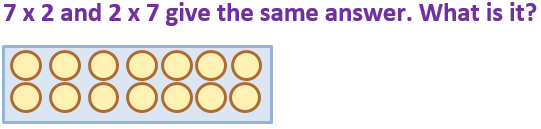72
12
27
14
2 groups of 7, or 7 groups of 2, makes 14
2.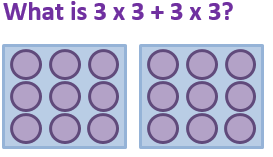18
9
99
81
3 x 3 = 9. 9 plus 9 = 18
3.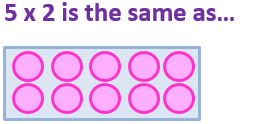2 + 2 + 2 + 2 + 2
4 x 5
5 + 5 + 5 + 5 + 5
5 x 5
5 x 2 is 5 groups of 2, or 2 + 2 + 2 + 2 + 2
4.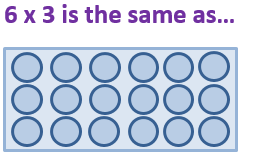3 + 3 + 3 + 3 + 3 + 3
6 + 6 + 6 + 6 + 6 + 6
3 x 3
6 x 6
6 x 3 is the same as 6 groups of 3, or 3 + 3 + 3 + 3 + 3 + 3
5.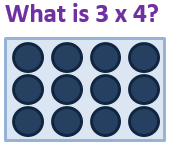18
12
8
14
3 groups of 4, or 4 + 4 + 4 = 12
6.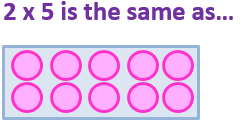2 + 5
4 x 5
5 + 5
5 x 5
2 x 5 is 2 groups of 5, or 5 + 5
7.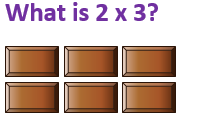6
8
4
3
2 groups of 3, or 3 + 3 = 6
8.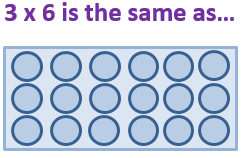3 + 3 + 3
6 + 6 + 6
3 x 3
6 x 6
3 x 6 is the same as 3 groups of 6, or 6 + 6 + 6
9.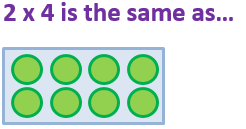2 + 2
4 x 2
4 + 4 + 4
2 x 2
2 groups of 4 is the same as 4 groups of 2
10.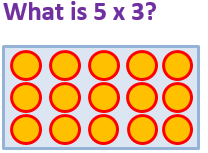30
13
26
15
5 groups of 3, or 3 + 3 + 3 + 3 + 3 = 15
Author:  Angela Smith